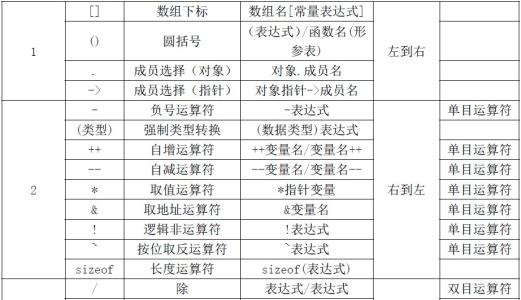# C中的逻辑运算符 “&&”、“||”、“！”是什么意思？怎么区分？1、“&&”（与）：逻辑与运算符

A=0;
B=0;
A&&B输出就是0

A=1;
B=0;
A&&B输出就是0

A=0;
B=1;
A&&B输出就是0

A=1;
B=1;
A&&B输出就是1

2、“||”（或）：逻辑或运算符

A=0;
B=0;
A||B输出就是0

A=1;
B=0;
A||B输出就是1

A=0;
B=1;
A||B输出就是1

A=1;
B=1;
A||B输出就是1

3、“！”（非）：逻辑非运算符
“非”，计算机中的非就是对值有相反的意思，!0=1，!1=0，它是单目操作符，也就是说只对他后面的值或表达式有影响。

A=0;
!A输出就是1

A=1;
!A输出就是0

A=1;
B=0；
!(A&&B)输出就是!(0)=1。

4、注意事项和简单例子

int  A=0，B=2，C=-1;
int  D=!A||(B&&C||A);

!A||(B&&C||A)
=!A||(1||A)       //括号里面运算符等级相同，所以从左到右
=!A||1           //选算括号里面的
=1||1                         //！优先级高于||
=1

### 联系我们